Factoring - Using an APP

 Using an "APP" (Application):

We are going to use an APP to find the roots of an equation, which in turn will let use establish the factors of the corresponding expression. This method is similar to what we saw under Factoring with the graphical connection. This will be a little faster as we do not have to actually produce the graph.

 NOTE: The displays below will show the screens from the TI-84 Plus CE with the complementary screen from the TI-84 Plus in a smaller size below. Both applications work in the same manner with only moderate differences in the displays.

On the TI-84 Plus CE (or the TI-84 PLus), under the APP key, you will find an application titled "PlySmlt2". This application is the "Polynomial Root Finder and Simultaneous Equation Solver". If you do not have this application, you can download it for free from at https://education.ti.com/en/software/search.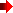NOTE: This APP will not work for polynomials of degree (order) greater than or equal to 4.
So we are talking about factoring degree 2 (quadratics) and degree 3 (cubics) polynomials only.

• Use 2nd QUIT to move between screens. Use option 6: QUIT APP to end the program.

QUESTION: Factor x2 - 12x + 36.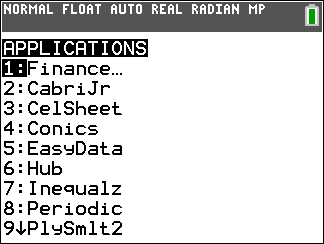Choose Option 9 PlySmlt2 (or option 4 below). The option "number" will vary.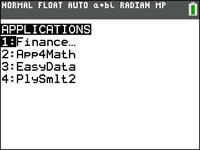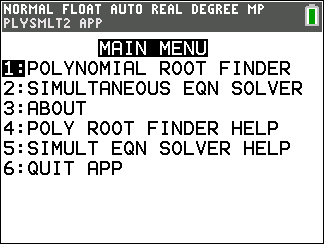Choose Option 1: Polynomial Root Finder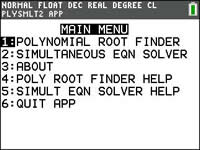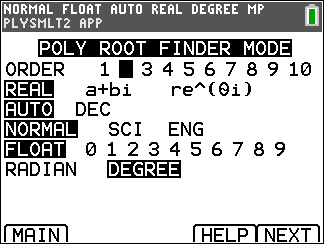Choose the ORDER (degree) of your polynomial. We are choosing ORDER 2. You can stay with REAL, AUTO, NORMAL, FLOAT and DEGREE.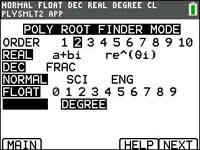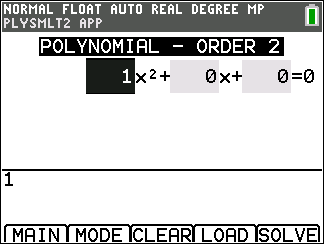Enter your coefficients. Use your ARROW key and ENTER.key You can change the + sign to negative, if needed. Hit SOLVE (using GRAPH key) when done entering.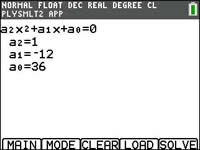. Be careful to enter "negative" and not "subtract".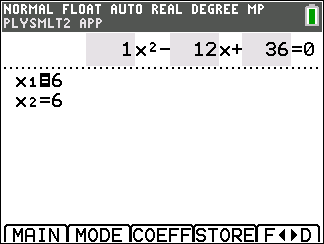If the polynomial has REAL roots, they will appear at this time.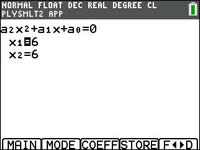Conclusion: Once you have the "roots" you can express the "factors". Root = 6 implies the Factor = (x - 6) So the factors are (x - 6)(x - 6).Finding Your Way Around TABLE of  CONTENTS